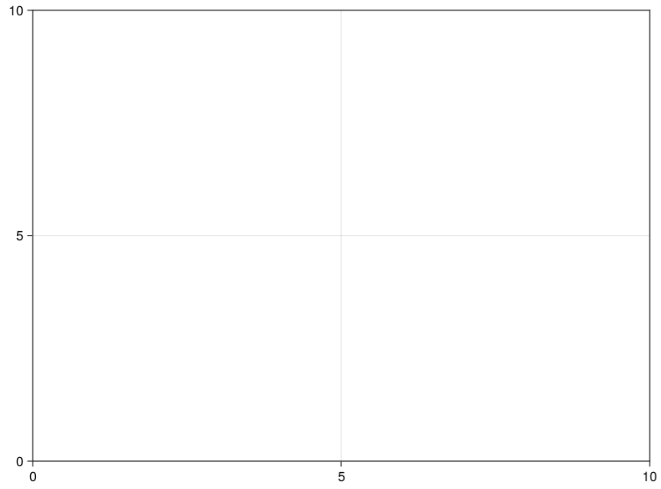# Text outside of Axis with Makie

Is it possible, using Makie.jl, to add text outside of an axis? For instance, in

``````using CairoMakie
fig = Figure()
ax = Axis(fig[1, 1])
``````I want to add text right on the top-left on the plot outside of the axis itself. I tried e.g.

``````text!(ax, -5, 10; text = L"\$ \$Upper")
``````

but of course that just changes the axis limits and puts it inside.

Something like this?

``````let
fig = Figure()
xs = 1:10
ax, plt = scatter(fig[1, 1], xs, xs.^2)
Label(fig[0, 0], "Test")
display(fig)
end
``````

There is also A LOT of detail on how to do this in the layout tutorial, where they go through, in complete detail on why and how, to make the following plot:

1 Like

Here are two options:

``````f = Figure()
Axis(f[1, 1], title = "Some text", titlealign = :left)
Axis(f[1, 2])
Label(f[1, 2, Top()], "Some Text", halign = :left)
f
``````

2 Likes